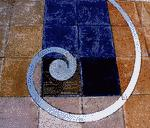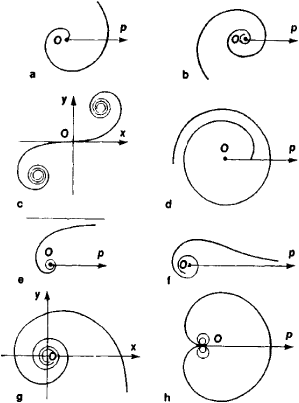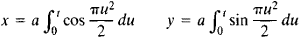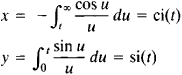spiral

(redirected from spiral worm)
Also found in: Dictionary, Thesaurus, Medical.

spiral

1. Geometry one of several plane curves formed by a point winding about a fixed point at an ever-increasing distance from it. Polar equation of Archimedes spiral: r = aθ; of logarithmic spiral: log r = aθ; of hyperbolic spiral: rθ = a, (where a is a constant)
2. another name for helix
3. a flight manoeuvre in which an aircraft descends describing a helix of comparatively large radius with the angle of attack within the normal flight range
4. Economics a continuous upward or downward movement in economic activity or prices, caused by interaction between prices, wages, demand, and production
Collins Discovery Encyclopedia, 1st edition © HarperCollins Publishers 2005

Spiral

Refers to forms that are generated by a continuous curve, traced by a point moving around a fixed point in a fixed plane, while steadily increasing the distance from that point.The following article is from The Great Soviet Encyclopedia (1979). It might be outdated or ideologically biased.

Spiral

a plane curve going around a certain point an infinite number of times and approaching or receding from the point on each circuit. If this point is chosen as the pole of a polar coordinate system, then the spiral is given by a popular equation p = f (φ) such that f (φ + 2π) > f (φ) or f (φ + 2π) < f (φ) for all*. In particular, a spiral is obtained if f (φ) is a monotone increasing or decreasing positive function.Figure 1. (a) Spiral of Archimedes, (b) logarithmic spiral, (c) Cornu spiral, (d) parabolic spiral, (e) hyperbolic spiral, (f) lituus, (g) si-ci spiral, (h) cochleold

The equation of the spiral of Archimedes (Figure 1 ,a) has the simplest form: ρ = α<t>. In the third century B.C., this spiral was studied by the ancient Greek mathematician Archimedes, in his treatise On Spirals, in connection with the problems of trisecting an angle and squaring the circle. Archimedes found the area bounded by an arc of the spiral extending from the pole to a certain point and by the line joining the pole and this point. This achievement was one of the first examples of the quadrature of a curvilinear region. The spiral of Archimedes is the pedal curve (seePEDAL CURVE) of the involute of a circle. This fact is made use of in certain drawbridge designs in balancing the variable tension of the chain. A cardioid eccentric cam—that is, an eccentric cam bounded by two arcs of Archimedean spirals—transforms uniform rotational motion into uniform translational motion. The distance between diametrically opposite points on the periphery of the cam is constant. The French mathematician P. de Fermat studied the generalized spiral of Archimedes (ρ/α)n = (Φ/2π)m and found the area bounded by an arc of the spiral extending from the pole to a certain point and by the line joining the pole and this point.

The equation ρ = ae defines the logarithmic, or equiangular, spiral (Figure l,b). The logarithmic spiral intersects all radius vectors drawn from the pole at the same angle α, where cot α = k. This property of the logarithmic spiral is made use of in designing rotary cutters in order to ensure a constant cutting angle. The logarithmic spiral is also encountered in the theory of spiral cases for hydraulic turbines. The theory of gear wheels makes use of the following property: two equal logarithmic spirals rotating about their poles can roll along each other without slipping. Gear trains with variable gear ratios can be obtained in this way.

In the stereographic projection of a sphere onto a plane, a logarithmic spiral is the projection of a loxodrome, which is a curve that cuts all meridians at the same angle. The Italian mathematician E. Torricelli determined the length of an arc of a logarithmic spiral: the arc length is proportional to the difference between the lengths of the radius vectors of the end points of the arc; more precisely, the arc length is (ρ2 ρ1))/cos α. The Swiss scientist J. Bernoulli showed that the evolute and caustic of a logarithmic spiral are logarithmic spirals. When a logarithmic spiral is rotated about the pole, the curve obtained is homothetic to the original curve. Under inversion with respect to a circle, a logarithmic spiral is transformed into a logarithmic spiral.

Another spiral of practical importance is the Cornu spiral, or clothoid, which is used in the graphic solution of certain problems in diffraction (Figure 1 ,c). The parametric equations of this spiral have the formThe Cornu spiral is an ideal transition curve for use in railroad tracks, since the spiral’s radius of curvature increases in proportion to the arc length.

There exist spirals that are involutes of closed curves. An example is the involute of a circle. The names of some spirals stem from the resemblance of the spirals’ polar equations to the equations of curves in Cartesian coordinates. For example, the parabolic spiral (Figure 1 ,d) has the equation (a – ρ)2 = , and the hyperbolic spiral (Figure l,e) has the equation ρ = α/φ. Other spirals include the lituus (Figure 1 ,f), whose equation is ρ2 = α/Φ, and the si-ci spiral (Figure 1 ,g), whose parametric equations have the formwhere si(t) and ci(i) are known as the sine integral and cosine integral, respectively. The curvature of this spiral is an exponential function of the arc length. Such spirals are used in french curves.

Reminiscent of a spiral is the cochleoid (Figure l,h), whose equation is φ = (α sin φ)/φ. This curve passes through the pole an infinite number of times, and each succeeding loop lies within the preceding loop.

Spirals are encountered in the study of singularities of differential equations (seeSINGULAR POINT).

Space curves that make an infinite number of circuits about an axis, for example, the helix, are also sometimes referred to as spirals.

What does it mean when you dream about a spiral?

Spiraling, either upward or downward, is often used figuratively to refer to the rapid rise and fall of finances. It may also symbolize flux in weather, health, and employment cycles, to name just a few. It can also simply mean that the dreamer feels things are “spiraling out of control.”

spiral

[′spī·rəl]
(mathematics)
A simple curve in the plane which continuously winds about itself either into some point or out from some point.
McGraw-Hill Dictionary of Scientific & Technical Terms, 6E, Copyright © 2003 by The McGraw-Hill Companies, Inc.

spiral

A continuously wound reinforcement in the form of a cylindrical helix.
McGraw-Hill Dictionary of Architecture and Construction. Copyright © 2003 by McGraw-Hill Companies, Inc.

spiral

A maneuver in which an airplane descends rapidly in a steeply banked turning flight path. The angle of attack remains within the normal range in a spiral. The aircraft speed tends to increase unless other-wise controlled by actions such as throttling back and/or using airbrakes.